# Advanced Geometry : How to find transformation for an analytic geometry equation

## Example Questions

### Example Question #4 : How To Find Transformation For An Analytic Geometry Equation

If g(x) is a transformation of f(x) that moves the graph of f(x) four units up and three units left, what is g(x) in relation to f(x)?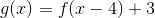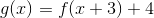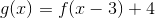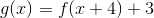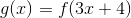Explanation:

To solve this question, you must have an understanding of standard transformations. To move a function along the x-axis in the positive direction, you must subtract the value from the operative x-value. For example, to move a function, f(x), five units to the left would be f(x+5).

To shift a function along the y-axis in the positive direction, you must add the value to the overall function. For example, to move a function, f(x), three units up would be f(x)+3.

The question asks us to move the function, f(x), left three units and up four units. f(x+3) will move the function three units to the left and f(x)+4 will move it four units up.

Together, this gives our final answer of f(x+3)+4.

### Example Question #1 : Transformation

Let f(x) = -2x2 + 3x - 5. If g(x) represents f(x) after it has been shifted to the left by three units, and then shifted down by four, which of the following is equal to g(x)?

-2x^2 + 15x - 36
-2x^2 - 9
-2x^2 - 9x - 18
-2x^2 + 3x - 12
-2x^2 + 31x - 124
Correct answer: -2x^2 - 9x - 18
Explanation:

We are told that g(x) is found by taking f(x) and shifting it to the left by three and then down by four. This means that we can represent g(x) as follows:

g(x) = f(x + 3) - 4

Remember that the function f(x + 3) represents f(x) after it has been shifted to the LEFT by three, whereas f(x - 3) represents f(x) after being shifted to the RIGHT by three.

f(x) = -2x2 + 3x - 5

g(x) =  f(x + 3) - 4 = [-2(x+3)2 + 3(x+3) - 5] - 4

g(x) = -2(x2 + 6x + 9) + 3x + 9 - 5 - 4

g(x) = -2x2 -12x -18 + 3x + 9 - 5 - 4

g(x) = -2x- 9x - 18 + 9 - 5 - 4

g(x) = -2x- 9x - 18

The answer is -2x- 9x - 18.

### Example Question #2 : Transformation

Let f(x) be a function. Which of the following represents f(x) after it has been reflected across the x-axis, then shifted to the left by four units, and then shifted down by five units?

–f(x – 4) – 5

–f(x – 5) – 4

–f(x + 4) – 5

f(x + 4) – 5

–f(x + 5) – 4

–f(x + 4) – 5

Explanation:

f(x) undergoes a series of three transformations. The first transformation is the reflection of f(x) across the x-axis. This kind of transformation takes all of the negative values and makes them positive, and all of the positive values and makes them negative. This can be represented by multiplying f(x) by –1. Thus, –f(x) represents f(x) after it is reflected across the x-axis.

Next, the function is shifted to the left by four. In general, if g(x) is a function, then g(x – h) represents a shift by h units. If h is positive, then the shift is to the right, and if h is negative, then the shift is to the left. In order, to shift the function to the left by four, we would need to let h = –4. Thus, after –f(x) is shifted to the left by four, we can write this as –f(x – (–4)) = –f(x + 4).

The final transformation requires shifting the function down by 5. In general, if g(x) is a function, then g(x) + h represents a shift upward if h is positive and a shift downward if h is negative. Thus, a downward shift of 5 to the function –f(+ 4) would be represented as –f(+ 4) – 5.

The three transformations of f(x) can be represented as –f(+ 4) – 5.

The answer is –f(+ 4) – 5.

### Example Question #3 : Transformation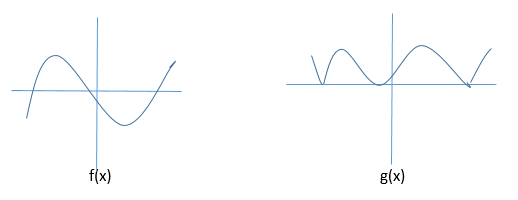The graphs of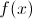and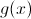are shown above. Which equation best describes the relationship betweenand?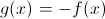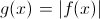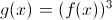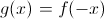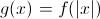Explanation: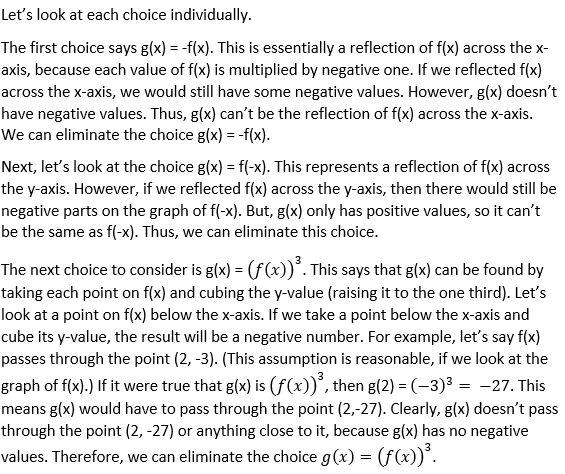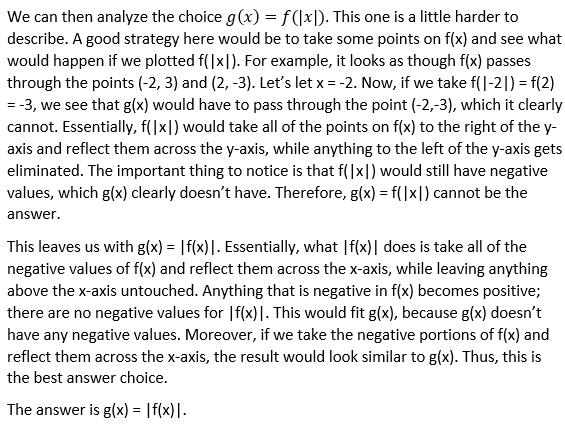### Example Question #221 : Coordinate Geometry

If the point (6, 7) is reflected over the line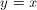and then over the x-axis, what is the resulting coordinate?

(7, 6)

(6, –7)

(–6, –7)

(6, 7)

(7, –6)

(7, –6)

Explanation:

A reflection over the lineinvolves a switching of the coordinates to get us (7, 6). A reflection over the x-axis involves a negation of the y-coordinate. Thus the resulting point is (7, –6).

### Example Question #5 : Transformation

You are looking at a map of your town and your house is located at the coordinate (0,0). Your school is located at the point (3,4). If each coordinate distance is 1.3 miles, how far away is your school?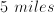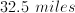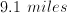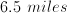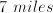Explanation:

The coordinate length between you and your school is equivalent to the hypotenuse of a right triangle with sides of 3 and 4 units: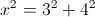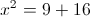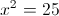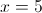The distance is 5 coordinate lengths, and each coordinate length corresponds to 1.3 miles of distance, so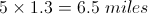### Example Question #6 : Transformation

Line m passes through the points (–4, 3) and (2, –6). If line q is generated by reflecting m across the line y = x, then which of the  following represents the equation of q?

2x + 3y = –6

3x + 2y = 18

–2x + 3y = 6

3x + 2y = 6

2x + 3y = 6

2x + 3y = –6

Explanation:

When a point is reflected across the line y = x, the x and y coordinates are switched. In other words, the point (a, b) reflected across the line y = x would be (b, a).

Thus, if line m is reflected across the line y = x, the points that it passes through will be reflected across the line y = x. As a result, since m passes through (–4, 3) and (2, –6), when m is reflected across y = x, the points it will pass through become (3, –4) and (–6, 2).

Because line q is a reflection of line m across y = x, q must pass through the points (3, –4) and (–6, 2). We know two points on q, so if we determine the slope of q, we can then use the point-slope formula to find the equation of q.

First, let's find the slope between (3, –4) and (–6, 2) using the formula for slope between the points (x1, y1) and (x2, y2).slope = (2 – (–4))/(–6 –3)

= 6/–9 = –2/3

Next, we can use the point-slope formula to find the equation for q.

y – y1 = slope(x – x1)

y – 2 = (–2/3)(x – (–6))

Multiply both sides by 3.

3(y – 2) = –2(x + 6)

3y – 6 = –2x – 12

2x + 3y – 6 = –12

2x + 3y = –6

The answer is 2x + 3y = –6.

### Example Question #221 : Coordinate Geometry

Explain in words the transformations from the parent function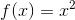for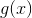below.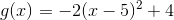Reflection over the x-axis, vertical stretch by a factor of 2, translate right 5 spaces and up 4 spaces.

Reflection over the x-axis, vertical stretch by a factor of 2, translate left 5 spaces and up 4 spaces.

Reflection over the y-axis, horizontal stretch by a factor of 2, translate left 5 spaces and up 4 spaces.

Reflection over the y-axis, vertical stretch by a factor of 2, translate right 5 spaces and up 4 spaces.

Reflection over the x-axis, vertical stretch by a factor of 2, translate right 5 spaces and up 4 spaces.

Explanation:

This quadratic is presented in vertex form.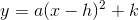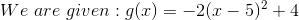First, the negative sign will reflect the graph over the x-axis, if the negative sign were inside the parentheses it would be reflected over the y-axis.

Second, the 2 will stretch the graph vertically, if the 2 had been inside the parentheses that would have been a horizontal shrink.

Third, the 5 inside the parentheses, translates the graph 5 spaces right because we are plugging in a positive 5 into the original vertex form for "h". A negative 5 would change the sign inside the parentheses.

Finally, the 4 outside the parentheses is the k-value, which translates the graph up 4 spaces in this case.

### Example Question #222 : Coordinate Geometry

If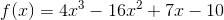, what wouldbe if it is equivalent toreflected over the x-axis.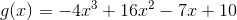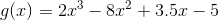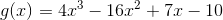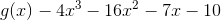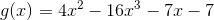Explanation:

If the function is reflected over the x-axis, then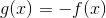.

It will be the opposite of what is currently written.

Therefore,becomes.

### Example Question #223 : Coordinate Geometry

What would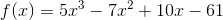become if it was reflected over the y-axis to become?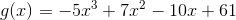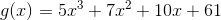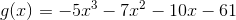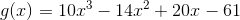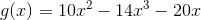Explanation:

When a function is reflected over the y-axis,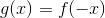becomes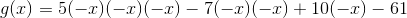which is simplifed as.